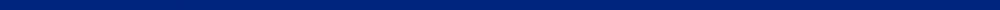CIVL 1101 - Computational AssignmentsHelpful links: Overview of Homework and Excel homework formats and a list of acceptable Abbreviations of Units.

 Assignment # Problems Due Format Information 0 CATME Team Making Survey First lab 8/25,  8/26, or 8/27 (W     R      F) None 1 Estimating error and significant figures - Solution 9/7 Homework 2 Leveling notes spreadsheet - Solution 9/14 Homework and Excel 3 Contour map problem - Solution 9/21 Homework and Excel 4 Mechanics of materials problems - Solution 9/28 Homework 5 Concrete mix design problem - Solution 10/5 Homework 6 Concrete mix design spreadsheet 10/14 Excel 7 Graphing with Excel 10/21 Excel 8 Mechanical analysis of soil - Solution 10/26 Homework 9 Sieve analysis spreadsheet - Solution 11/2 Excel 10 Filter efficiency calculator 11/9 Excel 11 Filter backwash calculations - Solution 11/16 Homework Bonus 11/30 No Format Required